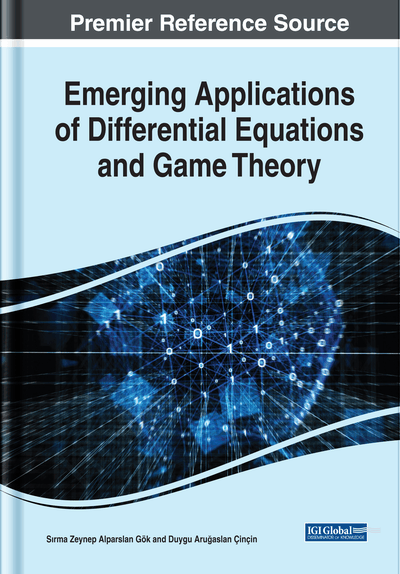# The Parseval Equality and Expansion Formula for Singular Hahn-Dirac System

Bilender P. Allahverdiev (Süleyman Demirel University, Turkey) and Hüseyin Tuna (Mehmet Akif Ersoy University, Turkey)
DOI: 10.4018/978-1-7998-0134-4.ch010

## Abstract

This work studies the singular Hahn-Dirac system given by Here 𝜇 is a complex spectral parameter, p(.) and r(.) are real-valued continuous functions at 𝜔0, defined on [𝜔0,∞) and q∈(0,1), , 𝜔>0, x∈[𝜔0,∞). The existence of a spectral function for this system is proved. Further, a Parseval equality and an expansion formula in eigenfunctions are proved in terms of the spectral function.
Chapter Preview
Top

## Introduction

The theory of Hahn difference operator was introduced by Hahn in 1949; see the papers (Hahn, 1949,1983). This operator provides a unifying structure for the study of the forward difference operator defined by

and the study of the quantum q-difference operator (Jackson, 1910) defined by

Recently, Hahn difference operators are receiving an increase of interest due to their applications in the construction of families of orthogonal polynomials and approximation problems (see Alvarez-Nodarse, 2006; Dobrogowsa & Odzijewicz, 2006; Kwon, Lee, Park & Yoo, 1998; Lesky, 2005; Petronilho, 2007 and the references therein).

In the literature there exist some papers studying the Hahn difference operator. The theory of linear Hahn difference equations was developed in the paper (Hamza & Ahmed, 2013). In (Hamza & Ahmed, 2013), the authors also study the existence and uniqueness of the solution for initial value problems related to Hahn difference equations. Hamza and Makharesh (Hamza & Makheresh, 2016) investigated Leibniz's rule and Fubini's theorem associated with the Hahn difference operator. The nonlocal boundary value problem for nonlinear Hahn difference equation was developed in the paper (Sitthiwirattham, 2016). In 2018, (Annaby, Hamza & Makherseh, 2018), the regular Hahn-Sturm-Liouville problem

has been studied, whereand v(.) is a real-valued continuous function at ω0, defined on [ω0,b]. Annaby et al. (Annaby, Hamza & Makherseh, 2018) defined a Hilbert space of ω,q- square summable functions. The authors discussed the formulation of the self-adjoint operator and the properties of the eigenvalues and the eigenfunctions. Furthermore, the authors constructed the Green's function and gave an eigenfunction expansion theorem. This equation is reduced to classic Sturm-Liouville problem when ω→0 and q→1. The French mathematicians Sturm and Liouville were introduced first this problem in 1837 (Sturm & Liouville, 1837). Since then this field is an active field of research. For a deeper discussion of this theory we refer the reader to (Zettl, 2005; Amrein, Hinz & Pearson, 2005; Mukhtarov & Aydemir, 2018; Aydemir, Olgar, Mukhtarov & Muhtarov, 2018; Olgar, Mukhtarov & Aydemir, 2018; Aydemir & Mukhtarov, 2017; Allahverdiev, Eryılmaz & Tuna, 2017; Tuna, 2014, 2016; Tuna & Özek, 2017; Tuna & Eryılmaz, 2013).

## Complete Chapter List

Search this Book:
Reset